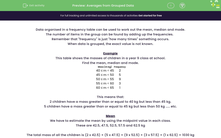# Find Averages from Grouped Data

In this worksheet, students will read grouped data from frequency tables in order to work out the mean, median and mode.Key stage:  KS 3

Curriculum topic:   Statistics

Curriculum subtopic:   Understand Variables, Representation, Measures and Spread

Difficulty level:#### Worksheet Overview

Data organised in a frequency table can be used to work out the mean, median and mode.

The number of items in the group can be found by adding up the frequencies.

Remember that 'frequency' is just 'how many times' something occurs.

When data is grouped, the exact value is not known.

Example

This table shows the masses of children in a Year 8 class at school.

Find the mean, median and mode.

Mass (m kg) Frequency
40 ≤ m < 45 2
45 ≤ m < 50 5
50 ≤ m < 55 9
55 ≤ m < 60 3
60 ≤ m < 65 1

This means that:

2 children have a mass greater than or equal to 40 kg but less than 45 kg.

5 children have a mass greater than or equal to 45 kg but less than 50 kg ..... etc.

Mean

We have to estimate the mean by using the midpoint value in each class.

These are 42.5, 47.5, 52.5, 57.5 and 62.5 kg

The total mass of all the children is (2 x 42.5) + (5 x 47.5) + (9 x 52.5) + (3 x 57.5) + (1 x 62.5) = 1,030 kg

There are 2 + 5 + 9 + 3 + 1 = 20 children in the class.

Mean mass = 1,030 ÷ 20 = 51.5 kg

Median

There are 20 children, so the 'middle person' will be the 10th and 11th.

The 10th and 11th person falls into the 50 ≤ m < 55 class.

The median class is 50 ≤ m < 55 kg

Mode

This is the class with the highest frequency.

The modal class is  50 ≤ m < 55 kg

Let's have a go at some questions now.

If you get stuck, you can click on the red button at the side of the screen and it will show you this introduction again.

### What is EdPlace?

We're your National Curriculum aligned online education content provider helping each child succeed in English, maths and science from year 1 to GCSE. With an EdPlace account you’ll be able to track and measure progress, helping each child achieve their best. We build confidence and attainment by personalising each child’s learning at a level that suits them.

Get started